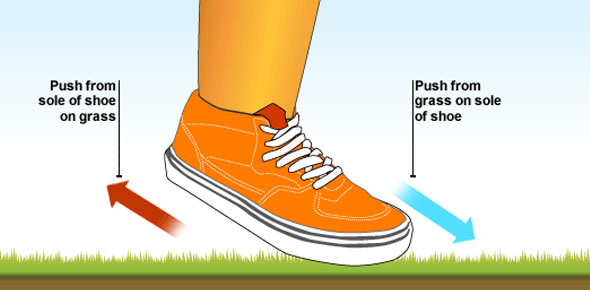# Chapter 2 B Investigating Motion

32 Questions | Attempts: 275Settings• 1.
The amount of matter in an object is its
• A.

Weight

• B.

Mass

• C.

Volume

• D.

Area

• 2.
If a planet has more gravity than earth, its mass is
• A.

Less than earth's mass

• B.

The same as earth's mass

• C.

More than earth's mass

• 3.
The farther away you get from earth, the less you feel the effect of its gravity.
• A.

True

• B.

False

• 4.
What causes an object to accelerate as it approaches the earth?
• A.

Friction

• B.

Gravity

• 5.
Acceleration occurs when an object
• A.

Goes faster

• B.

Slows down

• C.

Keeps a constant speed

• D.

Changes direction

• 6.
Friction can be reduced by adding
• A.

Sand

• B.

Oil

• C.

Wax

• D.

Wheels

• 7.
The force of friction moves in the same direction as the motion of an object.
• A.

True

• B.

False

• 8.
The force that resists the movement of one surface  past another surface is
• A.

Gravity

• B.

A push

• C.

A pull

• D.

Friction

• 9.
• A.

Increasing friction between your skin and the glass

• B.

Decreasing friction between your skin and the glass

• 10.
Friction caused by gas molecules in the atmosphere hitting an object and slowing it down is called
• A.

Streamlining

• B.

Air resistance

• 11.
Some ways to streamline an object  are to have
• A.

Rounded edges

• B.

less surface in the direction of motion

• C.

A large flat area in the front

• 12.
A meteorite burns up as it enters our atmosphere because of friction.
• A.

True

• B.

False

• 13.
What is the speed of a car that travels 120 miles in 4 hours?
• A.

60 miles per hour

• B.

30 kilometers per hour

• C.

30 miles per hour

• D.

60 kilometers per hour

• 14.
Forces act in pairs.
• A.

True

• B.

False

• 15.
The opposing force to an action force is
• A.

A reaction force

• B.

A reverse force

• 16.
What makes an object move or stop?
• A.

Acceleration

• B.

Inertia

• C.

Force

• 17.
When riding a skateboard, when you push your foot backwards against the floor, the skateboard
• A.

Moves backward

• B.

Moves forward

• C.

Stays in place

• 18.
The tendency of an object to stay still if it is at rest or to keep moving if it is moving is called
• A.

Gravity

• B.

Air resistance

• C.

Inertia

• D.

Acceleration

• 19.
If you run at a speed of 5 mph, how far would you run in 3 hours?
• A.

3 miles

• B.

15 miles

• C.

2 miles

• D.

8 miles

• 20.
Changing direction when you are moving changes your
• A.

Speed

• B.

Time

• C.

Gravity

• D.

Velocity

• 21.
The formula for speed is
• A.

Distance X time

• B.

Distance / time

• C.

Length X width

• 22.
The force that allows a parachute to fall safely to the ground is
• A.

Gravity

• B.

Air resistance

• C.

Streamlining

• 23.
Objects of different masses pull on each other equally, and the object with less mass
• A.

Moves toward the other object

• B.

Moves away from the other object

• C.

Stays still

• 24.
An object's weight
• A.

Is the same wherever it is.

• B.

Changes when gravity changes.

• 25.
If your mom puts her car on cruise control and travels at a constant speed of 65 mph, her acceleration is
• A.

Increasing

• B.

Decreasing

• C.

Zero

## Related TopicsBack to top
×

Wait!
Here's an interesting quiz for you.